# fcontour

•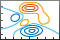## 语法

fcontour(f)
fcontour(f,xyinterval)
fcontour(___,LineSpec)
fcontour(___,Name,Value)
fcontour(ax,___)
fc = fcontour(___)

## 说明

fcontour(f) 根据 x 和 y 的默认区间 [-5 5] 和 z 的固定级别值绘制 z = f(x,y) 函数的等高线。

fcontour(f,xyinterval) 将在指定区间绘图。要对 x 和 y 使用相同的区间，请将 xyinterval 指定为 [min max] 形式的二元素向量。要使用不同的区间，请指定 [xmin xmax ymin ymax] 形式的四元素向量。
fcontour(___,LineSpec) 设置等高线的线型和颜色。例如，'-r' 指定红色线条。在前面的任何输入参数组合之后使用此选项。

fcontour(___,Name,Value) 使用一个或多个名称-值对组参数指定线条属性。
fcontour(ax,___) 将图形绘制到 ax 指定的坐标区中，而不是当前坐标区中。

fc = fcontour(___) 返回 FunctionContour 对象。可使用 fc 查询和修改特定 FunctionContour 对象的属性。有关属性列表，请参阅 FunctionContour 属性。

## 示例

$-5$-5 的默认区间绘制 $f\left(x,y\right)=\mathrm{sin}\left(x\right)+\mathrm{cos}\left(y\right)$ 的等高线。

f = @(x,y) sin(x) + cos(y); fcontour(f)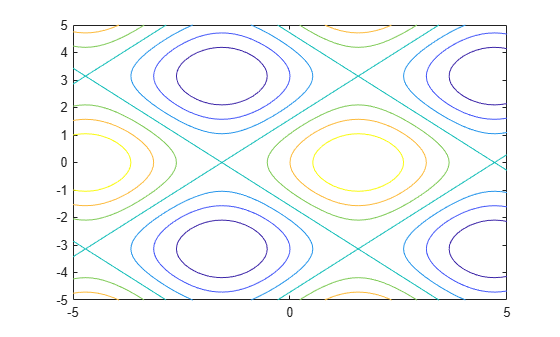$\begin{array}{cc}erf\left(x\right)+\mathrm{cos}\left(y\right)& -5

fcontour(@(x,y) erf(x) + cos(y),[-5 0 -5 5]) hold on fcontour(@(x,y) sin(x) + cos(y),[0 5 -5 5]) hold off grid on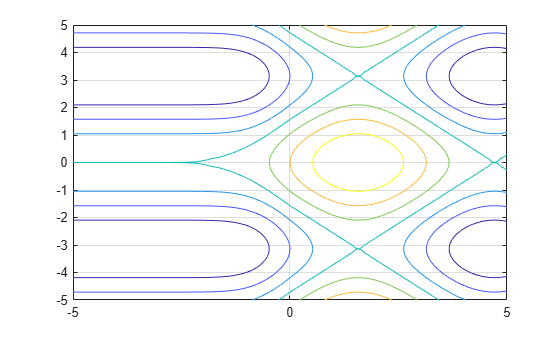f = @(x,y) x.^2 - y.^2; fcontour(f,'--','LineWidth',2)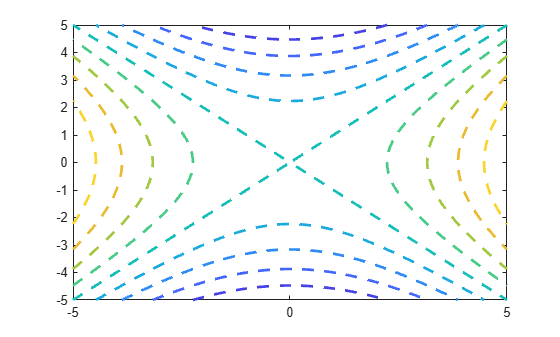fcontour(@(x,y) sin(x)+cos(y)) hold on fcontour(@(x,y) x-y) hold off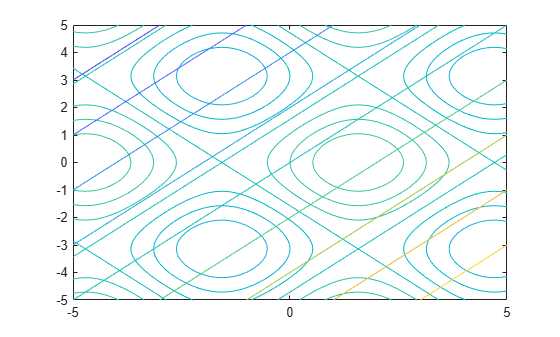f = @(x,y) exp(-(x/3).^2-(y/3).^2) + exp(-(x+2).^2-(y+2).^2); fc = fcontour(f)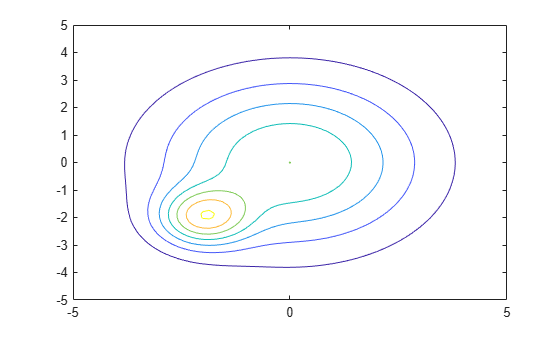fc = FunctionContour with properties: Function: @(x,y)exp(-(x/3).^2-(y/3).^2)+exp(-(x+2).^2-(y+2).^2) LineColor: 'flat' LineStyle: '-' LineWidth: 0.5000 Fill: off LevelList: [0.2000 0.4000 0.6000 0.8000 1 1.2000 1.4000] Use GET to show all properties 

fc.LineWidth = 1; fc.LineStyle = '--'; fc.LevelList = [1 0.9 0.8 0.2 0.1]; colorbar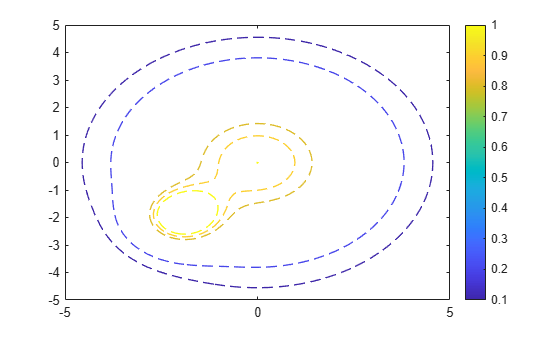$erf\left(\left(y+2{\right)}^{3}\right)-{e}^{\left(-0.65\left(\left(x-2{\right)}^{2}+\left(y-2{\right)}^{2}\right)\right)}.$

f = @(x,y) erf((y+2).^3) - exp(-0.65*((x-2).^2+(y-2).^2)); fcontour(f,'Fill','on');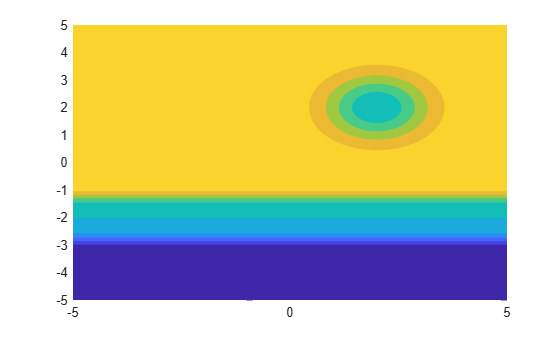f = @(x,y) sin(x) + cos(y); fcontour(f,'LevelList',[-1 0 1])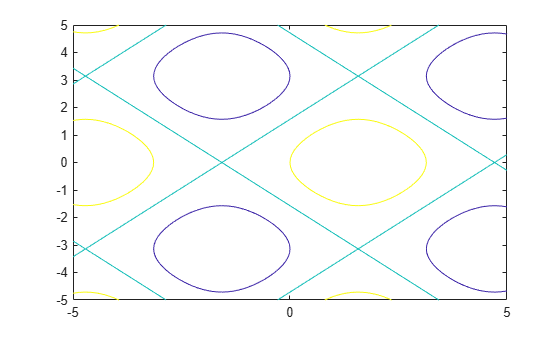f = @(x,y) sin(x).*sin(y); tiledlayout(2,1) nexttile fcontour(f) title('Default Mesh Density (71)') nexttile fcontour(f,'MeshDensity',200) title('Custom Mesh Density (200)')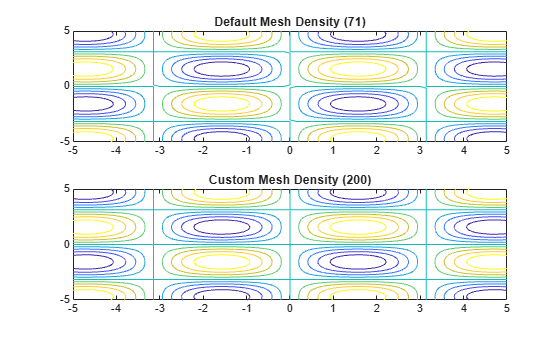fcontour(@(x,y) x.*sin(y) - y.*cos(x), [-2*pi 2*pi], 'LineWidth', 2); grid on title({'xsin(y) - ycos(x)','-2\pi < x < 2\pi and -2\pi < y < 2\pi'}) xlabel('x') ylabel('y')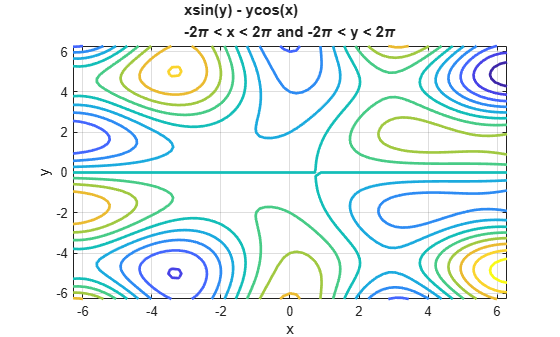ax = gca; ax.XTick = ax.XLim(1):pi/2:ax.XLim(2); ax.XTickLabel = {'-2\pi','-3\pi/2','-\pi','-\pi/2','0',... '\pi/2','\pi','3\pi/2','2\pi'}; ax.YTick = ax.YLim(1):pi/2:ax.YLim(2); ax.YTickLabel = {'-2\pi','-3\pi/2','-\pi','-\pi/2','0',... '\pi/2','\pi','3\pi/2','2\pi'};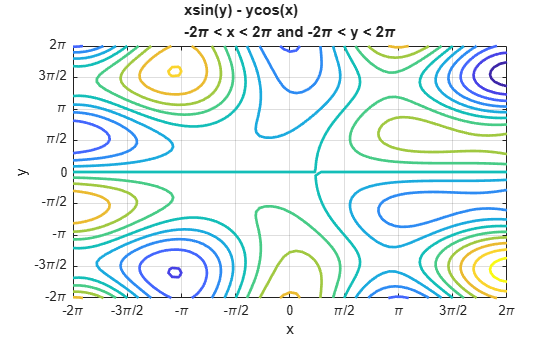## 输入参数

xy 的绘图区间，指定为下列形式之一：

• [min max] 形式的向量 - 对 xy 都使用区间 [min max]

• [xmin xmax ymin ymax] 形式的向量 - 对 x 使用区间 [xmin xmax]，对 y 使用区间 [ymin ymax]

-实线（默认）
--虚线
:点线
-.点划线

y

m

c

r

g

b

w

k

### 名称-值参数

• 值为 'on' 表示用颜色填充等高线之间的空间。

• 值为 'off' 表示不填充等高线之间的空间。

• RGB 三元组是包含三个元素的行向量，其元素分别指定颜色中红、绿、蓝分量的强度。强度值必须位于 [0,1] 范围内，例如 [0.4 0.6 0.7]

• 十六进制颜色代码是字符串标量或字符向量，以井号 (#) 开头，后跟三个或六个十六进制数字，范围可以是 0F。这些值不区分大小写。因此，颜色代码 "#FF8800""#ff8800""#F80""#f80" 是等效的。

"red""r"[1 0 0]"#FF0000""green""g"[0 1 0]"#00FF00""blue""b"[0 0 1]"#0000FF""cyan" "c"[0 1 1]"#00FFFF""magenta""m"[1 0 1]"#FF00FF""yellow""y"[1 1 0]"#FFFF00""black""k"[0 0 0]"#000000""white""w"[1 1 1]"#FFFFFF""none"不适用不适用不适用无颜色

RGB 三元组十六进制颜色代码外观
[0 0.4470 0.7410]"#0072BD"[0.8500 0.3250 0.0980]"#D95319"[0.9290 0.6940 0.1250]"#EDB120"[0.4940 0.1840 0.5560]"#7E2F8E"[0.4660 0.6740 0.1880]"#77AC30"[0.3010 0.7450 0.9330]"#4DBEEE"[0.6350 0.0780 0.1840]"#A2142F"